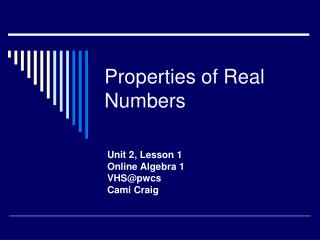# Properties of Real Numbers - PowerPoint PPT PresentationDownload PresentationProperties of Real Numbers

Properties of Real NumbersDownload Presentation## Properties of Real Numbers

- - - - - - - - - - - - - - - - - - - - - - - - - - - E N D - - - - - - - - - - - - - - - - - - - - - - - - - - -
##### Presentation Transcript

1. Properties of Real Numbers Unit 2, Lesson 1 Online Algebra 1 VHS@pwcs Cami Craig

2. Properties In this lesson we are going to look at properties, rules of mathematics that can be proven. We will be looking at properties of equality, and real number properties.

3. Properties of Real Numbers • Properties of Real Numbers include: • Commutative Property • Associative Property • Identity Property • Distributive Property • Inverse Property • Closure Property

4. Commutative Properties Commutative Properties deal with order. Order in multiplication and addition do not matter! Addition a + b = b + a Or 5 + 9 = 9 + 5 …..Is this true? Try it! Of course it is 14 = 14 Multiplication ab = ba Or 5(9) = 9(5) …..Is this true? Try it! Of course it is 45 = 45

5. Commutative Properties and Subtraction Does the Commutative Property hold for subtraction and division? Let’s try: If the commutative property holds for subtraction then the following should be true: 6 – 3 = 3 – 6 But we know it isn’t true 6 – 3 = 3 and 3 – 6 = -3 So the commutative property does NOT work for subtraction.

6. Commutative Properties and Division Does the Commutative Property hold for division? Let’s try: If the commutative property holds for division then the following should be true: 10 ÷ 5 = 5 ÷ 10 But we know it isn’t true 10 ÷ 5 = 2 and 5 ÷ 10 = 0.5 So the commutative property does NOT work for division.

7. Associative Properties Associative Properties deal with Grouping. Grouping in multiplication and addition do not matter! But just like the commutative properties the associative property does not apply to subtraction. Multiplication a(bc) = (ab)c Or 4 x (6 x 2) = (4 x 6) x 2 …..Is this true? Try it! 4 x (6 x 2) = (4 x 6) x 2 4 x 12 = 24 x 2 48 = 48 Addition a + (b + c) = (a + b) + c Or 11 +(5 + 9) = (11 + 5) + 9 …..Is this true? Try it! 11 + ( 5 + 9) = (11 + 5) + 9 11 + 14 = 16 + 9 25 = 25

8. Associative and Commutative Properties How can you tell these properties apart? Commutative Properties 8 + 11 = 11 + 8 4 x 5 = 5 x 4 Associative Properties 3 + ( 6 + 4) = (3 + 6) + 4 7 x (3 x 5) = (7 x 3) x 5 Notice that in the commutative property the order of the numbers change, while in the associative properties the order stays the same, but the grouping changes.

9. Distributive Property The distributive property is: a(b + c) = ab + ac Or 2( 4 + 5) = 2 x 4 + 2 x 5 I like to call the Distributive Property the fair share property, because the number on the outside of the parentheses is multiplied to both numbers in the parentheses.

10. Identity Properties The Identity Properties deal with getting back the same thing. Addition When we add 0 to a number, we get that original number back: For example: A + 0 = A -4 + 0 = -4 We actually call 0 the Additive Identity Element. Multiplication When we multiply 1 to a number, we get that original number back: For example: 1a = a -15(1) = -15 We actually call 1 the Multiplicative Identity Element.

11. Inverse and Closure Properties • The inverse property for addition states that a+ -a = 0. • The inverse property for multiplication states that a x 1/a = 1. • The closure property for addition states that a + b is a real number. • The closure property for multiplication states that a x b is a real number.

12. -2(3 + 4) = -2 x 3 + -2 x 4 5 + (3 + 6) = (3 + 6) + 5 5(1) = 5 17 x (8 x 2) = (17 x 8) x 2 9 + 0 = 9 4 x ¼ = 1 Distributive Property Commutative Property Notice that although there are parentheses, it is the order that changes not the grouping. Identity Property of Multiplication Associative Property Identity Property of Addition Inverse Property of Mult. Let’s see what you have learned so far!What property does each example represent?

13. Properties of Equality Properties of equality include the following: • The Reflexive Property • The Symmetric Property • The Transitive Property

14. Properties of Equality • Reflexive Property of Equality • a = a • -9 = -9 • Symmetric Property of Equality • If a = b, then b = a. • If 15x = 45, then 45 = 15x • Transitive Property of Equality • If a = b and b = c, then a = c • If d = 3y and 3y = 6, then d = 6.

15. 9 = 9 a + 8 = 8 + a If x + 8 = 9, and 9 = 4 + 5, then x + 8 = 4 + 5 3(x – 7) = 3x – 21 5 x 1 = 5 If 16 = 4x, then 4x = 16 7 + -7 = 0 Reflexive Commutative Transitive Distributive Identity Symmetric Inverse ReviewWhat property is each of the following an example of?